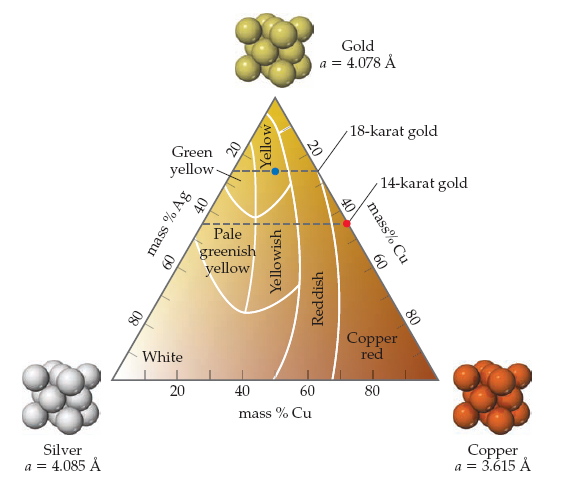⚠️Our tutors found the solution shown to be helpful for the problem you're searching for. We don't have the exact solution yet.

# Solution: The karat scale used to describe gold alloys is based on mass percentages. If an alloy is formed that is 50 mol% copper and 50 mol% gold, what is the karat number of the alloy? What is the color of this alloy?

###### Problem

The karat scale used to describe gold alloys is based on mass percentages. If an alloy is formed that is 50 mol% copper and 50 mol% gold, what is the karat number of the alloy? What is the color of this alloy?